## List of some known Runge-Kutta methods family (PRELIMINARY version)

Back to main page
Last update: 2013-02-07

Here you can find some (known) families of Runge-Kutta (RK) methods in Maple (text) file.
For example, classical RK-methods of order 4 is represented by next procedure:
```RK_44 := proc(c2, c3)::Matrix;
local a,d;
if c2=1/2 then ERROR(`RK_44: c2=1/2`); end if;
if c2=c3  then ERROR(`RK_44: c2=c3`);  end if;
if c2=1   then ERROR(`RK_44: c2=1`);   end if;
if c3=1   then ERROR(`RK_44: c3=1`);   end if;
d := factor(3-4*(c2+c3)+6*c2*c3);
if d=0 then ERROR(`RK_set44: 3-4*(c2+c3)+6*c2*c3=0`); end if;
a := Matrix(5,5);
a[2,1] := c2;
a[3,2] := c3*(c3-c2)/(2*c2*(1-2*c2));
a[3,1] := c3-a[3,2];
a[4,2] := (c2-1)*(2-c2-5*c3+4*c3*c3)/(2*c2*(c3-c2)*d);
a[4,3] := (1-2*c2)*(c3-1)*(c2-1)/(c3*(c3-c2)*d);
a[4,1] := 1 - a[4,3] - a[4,2];
a[5,2] := (1-2*c3)/(12*c2*(c2-1)*(c3-c2));
a[5,3] := (2*c2-1)/(12*c3*(c3-1)*(c3-c2));
a[5,4] := (3-4*c2-4*c3+6*c2*c3)/(12*(c2-1)*(c3-1));
a[5,1] := 1 - a[5,4] - a[5,3] - a[5,2];
simplify(a);
end proc;
```

Name psReferenceFeature
Order 4
RK_44(c2,c3) 44  Classical
RK_44a(b3) 44  c2=c3=1/2
RK_44b(b4) 44  c2=1, c3=1/2
RK_45(c2, c3, c4, c5, b2, a32, a42)45 ? dim = 7
Order 5
RK_56 (c2, c3, c4, c5, a43) 56  Butcher, b2=0
RK_56a(c2, c3, c4, c5, b2, a43) 56 ? dim=6
Order 6
RK_67(c2, c3, c5, c6) 67  Butcher, b2=0
RK_67a(c3, c5, c6) 67 ? b2<>0, b1=b7=1/12
RK_67b(c2, a32, b3) 67 ? b2<>0, c2=c3=c6
Order 7
RK_79(c4, c5) 79  Butcher, b2=b3=0

1. Butcher, J. C., "Numerical Methods for Ordinary Differential Equations" Second Edition, J. Wiley, Chichester, (2008)

### Methods of order 4

#### RK_44(c2,c3)

RK_44(c2,c3) - standart 4-stage RK method of order 4.
Restrictions: c2!=1/2, c2!=1, c3!=1, c3!=c2, 3-4(c2+c3)+6 c2 c3 !=0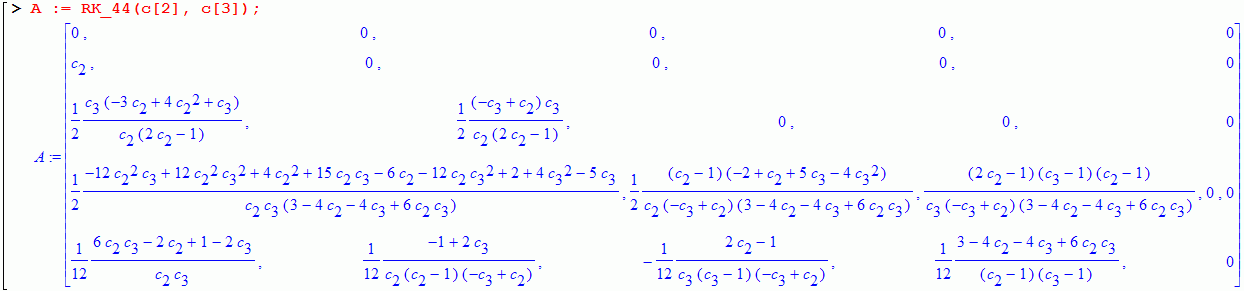#### RK_44a(b3)

c2=1/2, c3=1/2, c4=1.
Restrictions: b3!=0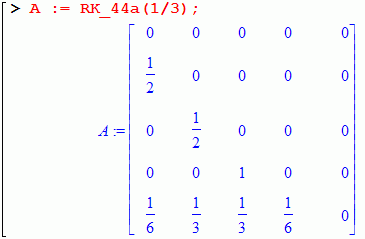#### RK_44b(b4)

c2=1, c3=1/2, c4=1.
Restrictions: b4!=0#### RK_45(c2, c3, c4, c5, b2, a32, a42)### Methods of order 5

#### RK_56 (c2, c3, c4, c5, a43)

(b2=0)!#### RK_56a(c2, c3, c4, c5, b2, a43)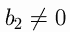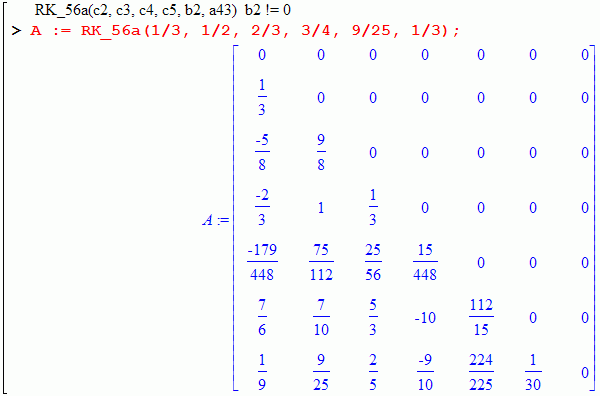Be carefull! Afterwe obtain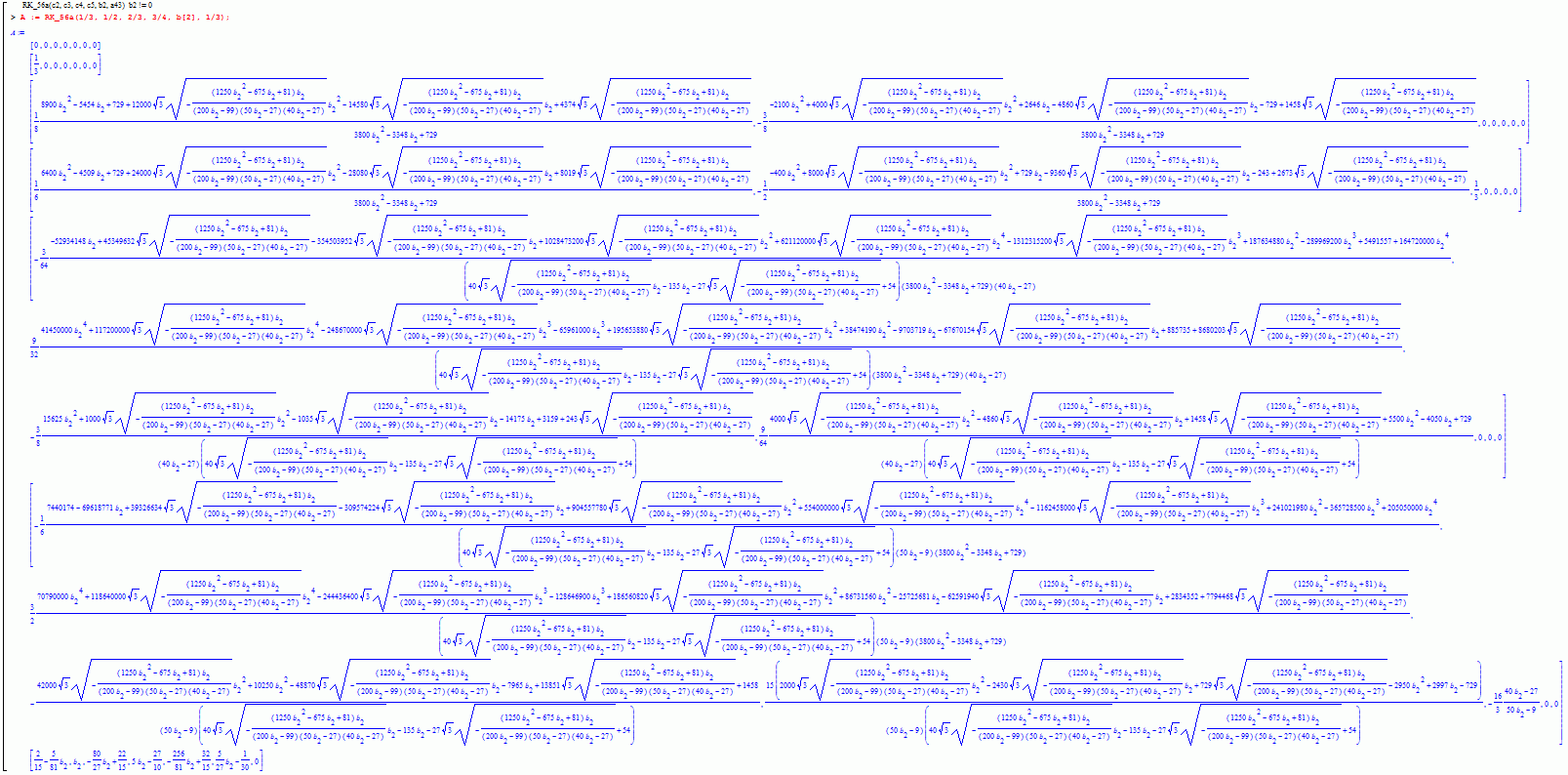#### RK_67(c2, c3, c5, c6)

(b2=0).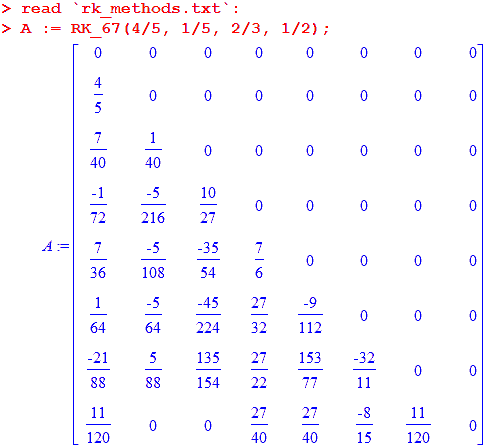#### RK_67a(c3, c5, c6)(b1=b7=1/12)
Let A be the extended matrix of the 7-stage RK-method of order 6, A is a lowertrianle matrix of size 8*8 with zero diagonal:As usual, lets introduce variables ci:Lets introduce variables d3, ..., d7 by formulas: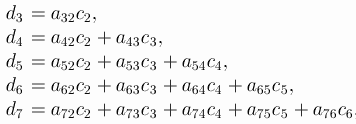In our case c7=1, d7=1/2.
Some of order conditions contains only bi, ci, di:for k,l > = 0, k+2l < p, orLets introduce variables e3, ..., e7 by formulas: ei=di-ci2/2:(e7=0). So: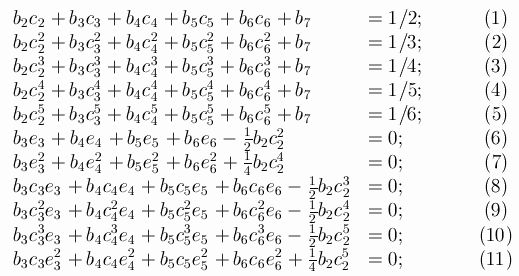One can express variables e3,e4,e5,e6 from equations (6,8,9,10):and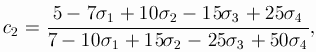where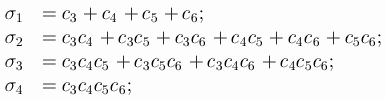Thenwhereandis elementary symmetric functions of variables x1, x2, x3.
With the substitution of found solutions in the complete system of orders conditions, it turns out that not every set of the above the free variables (c2,c3,c4,c5,c6), there is a solution of the full system. More exactly: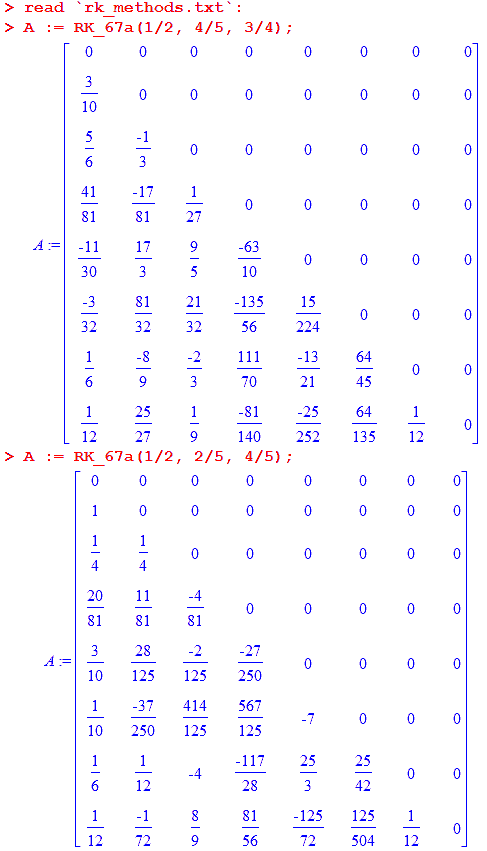#### RK_67b(c2, a32, b3)(c2=c3=c6)
c4 = c2/(15*c2^2 - 10*c2 + 2);
c5 = (9*c2-4)/(3*(5*c2-2));#### RK_79(c4, c5)

Methods from J.Butcher's book (see reference above), page 178.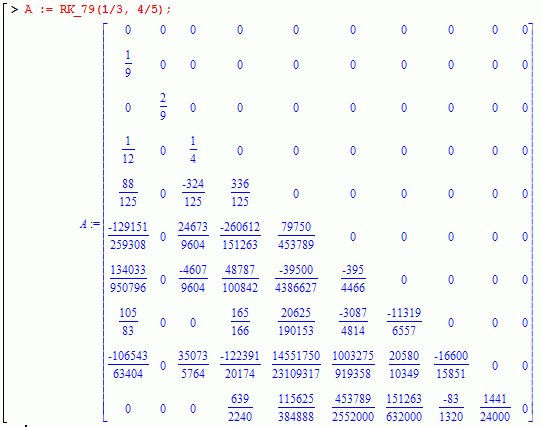You may also use:or even:,
but be careful!

Main page